# OECD Discriminatory family code data analysis 8 - Comparing some classification methods.Photo by Marek Piwnicki on Unsplash

This post is following of above post.

In this post, I will do some classification methods.

Firstly, I make binary variable.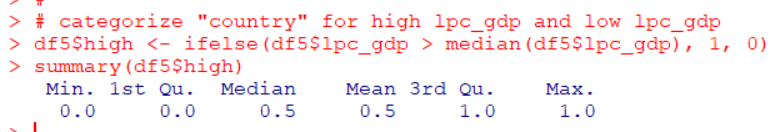I made a binary variable named high, that shows 1 when lpc_gdp is hgher than the mdeian and 0 when lower than median.

Before making models, I load caret package/

Then, I make LPM: Linear Probability Model, which is liear regression model with OLS estimate.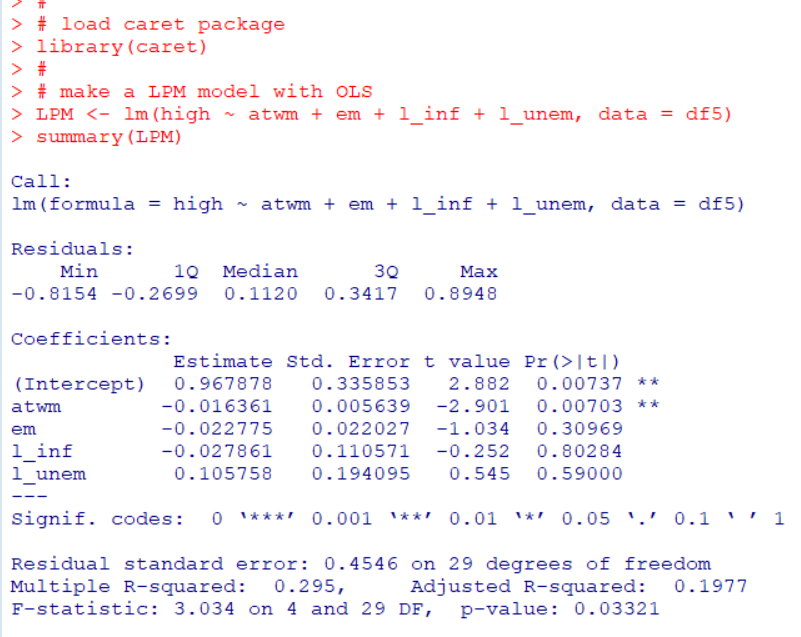"atwm": Attitides Towards Working Mother is only significant variable.

Then, make a prediction and calculating how much prediction is correct.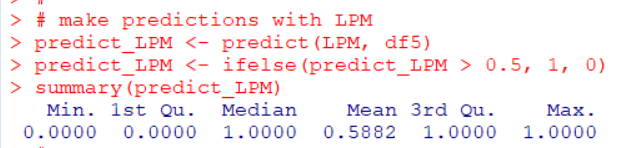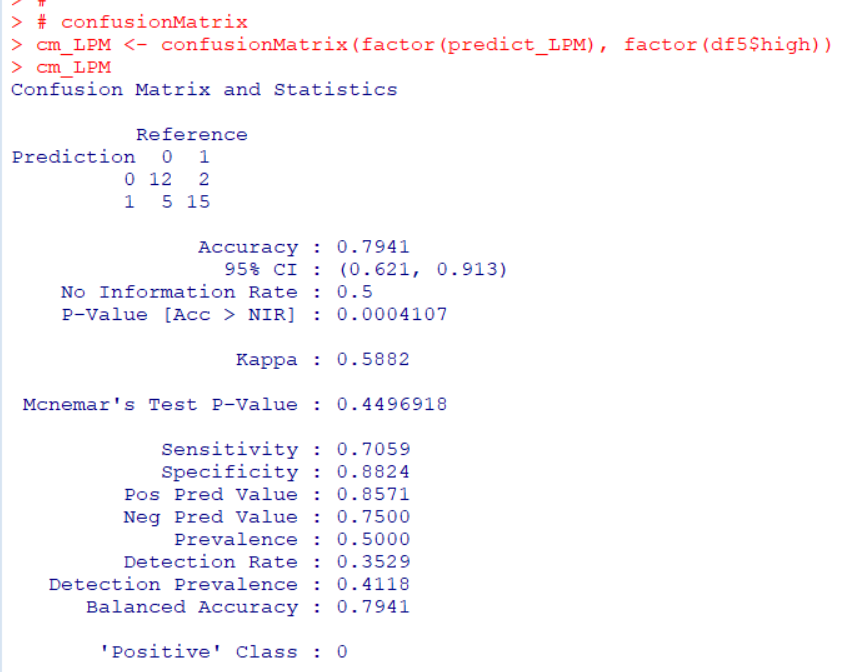Accuracy is 0.7941. This means 79.4% is correctly predicted.

Next, I use SVM: Support Vector Machine. I use e1071 package svm() function.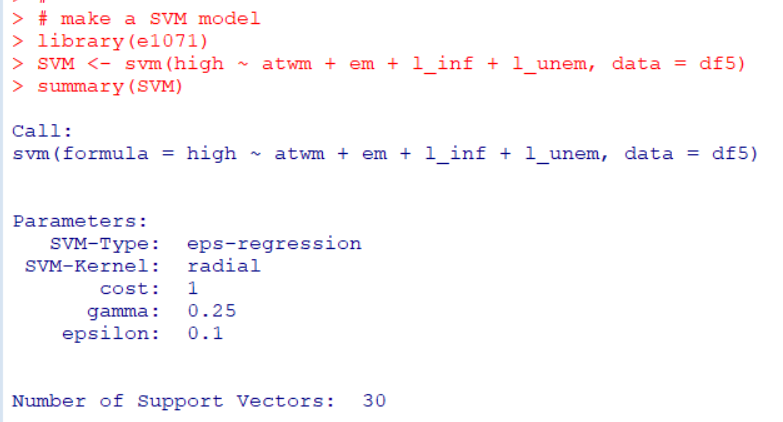with SVM model, what is accuracy?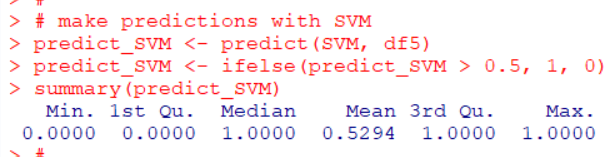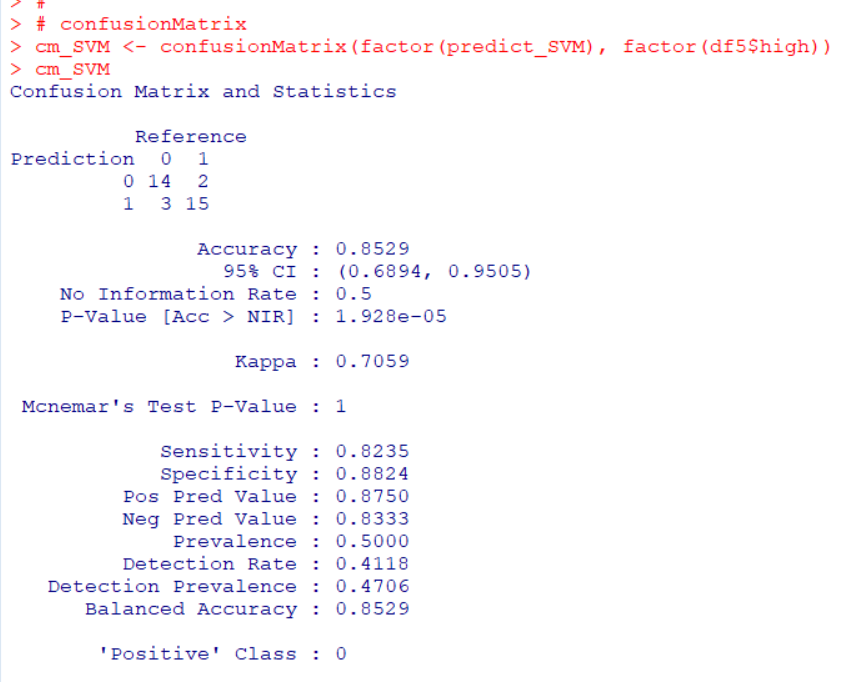Accuracy is 0.8529. So SVM is betther than LPM.

Next, I use GAM: Generalized Additive Model. I use gam() function in mgcv package.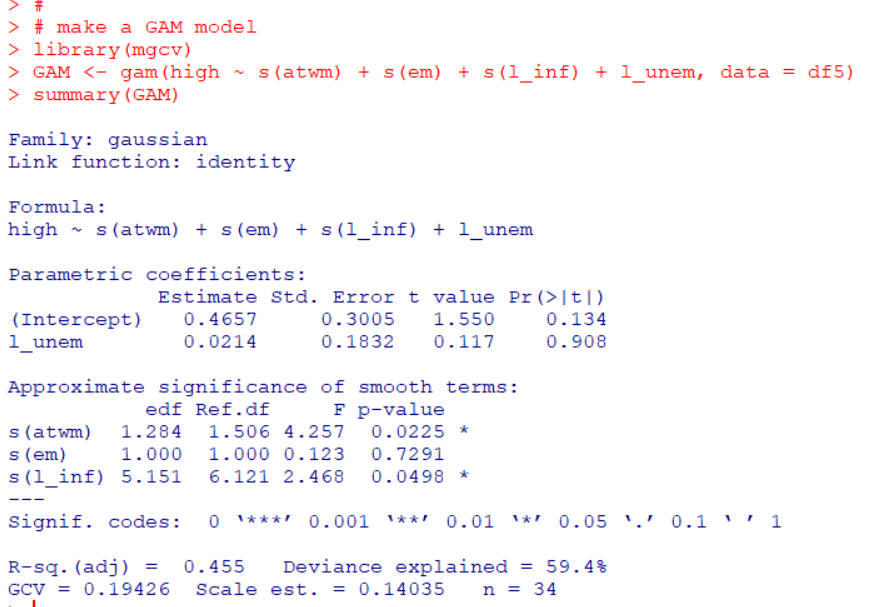In GAM, s(atwm) and s(l_inf) are significant variables.

Let's plot GAM model.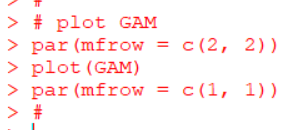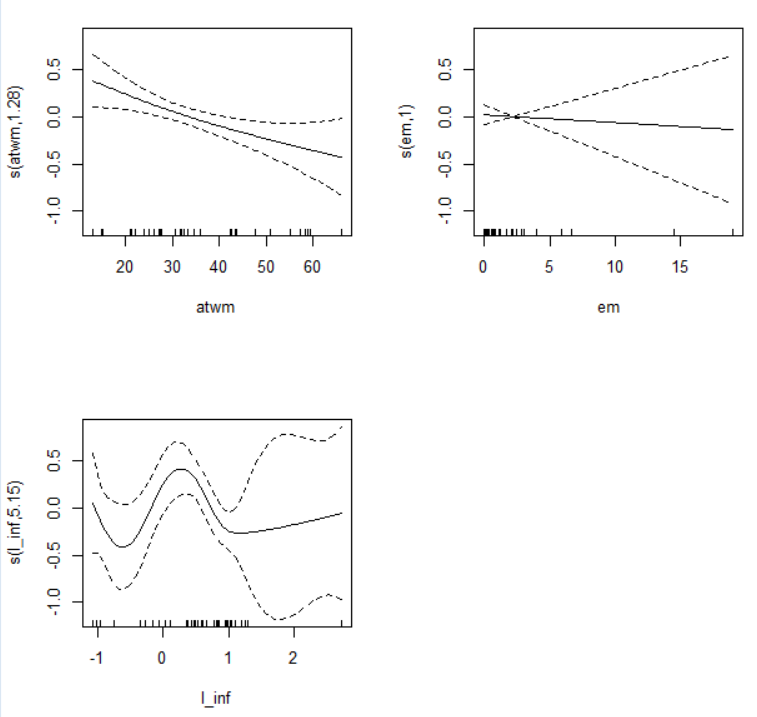The plot shows s(em) is not needed s().
So, let' make another GAM model, this time s(l_unem).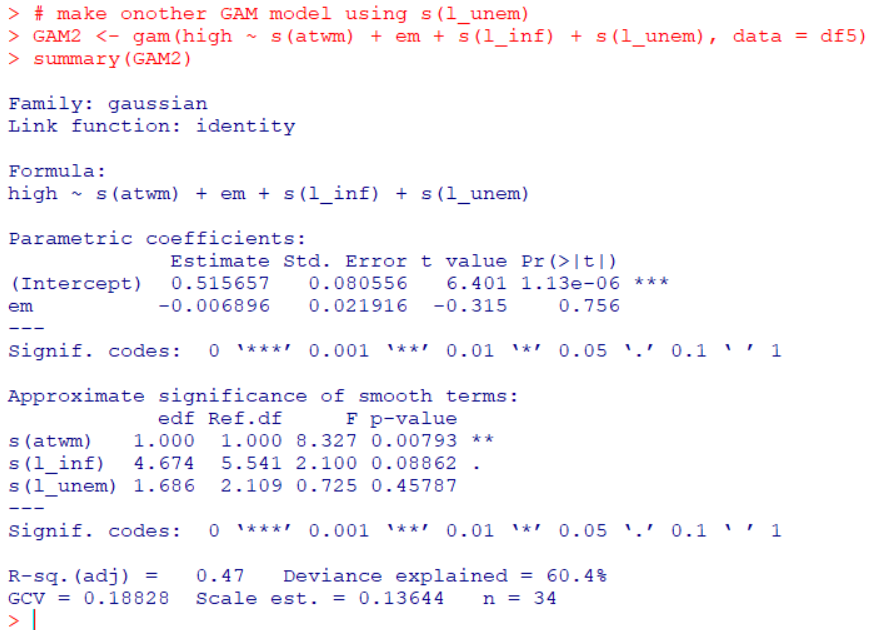plot GAM2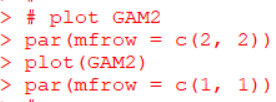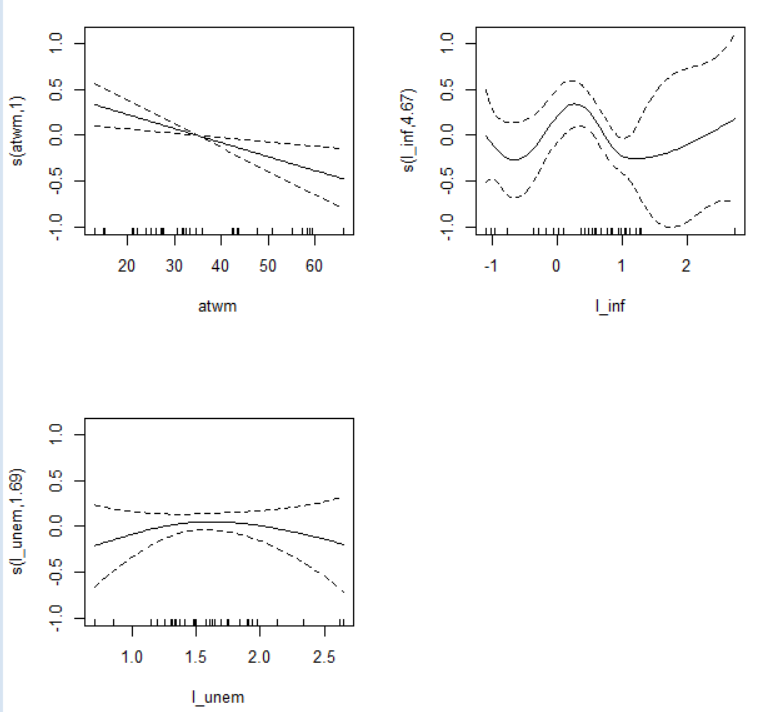Let's make predictions with GAM model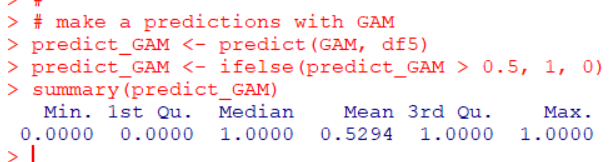Let's get accuracy og GAM.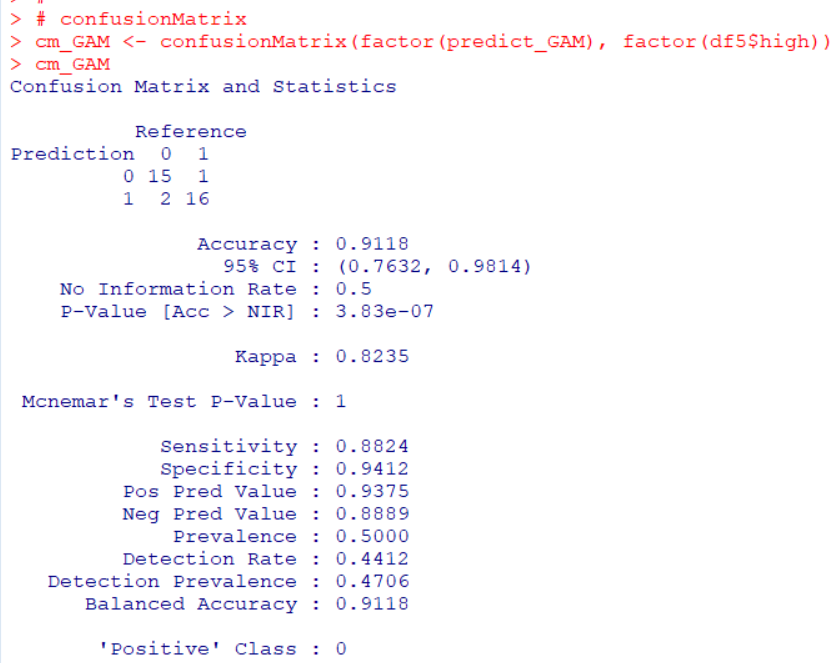Accuracy us 0.9118.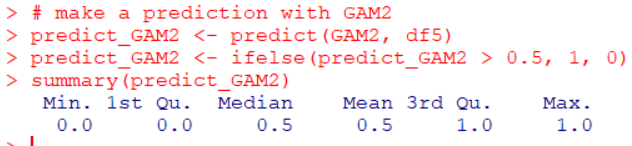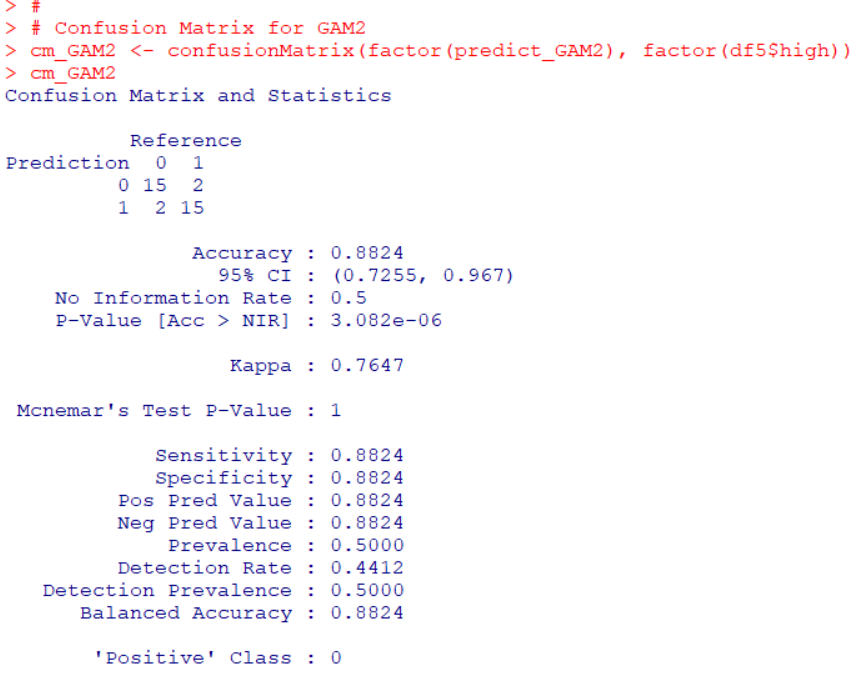Accuracy is 0.8824. So, the fist GAM model is better.

Next, I use tree model.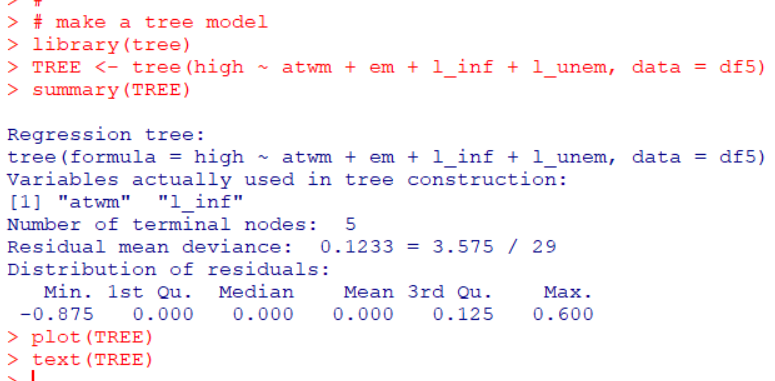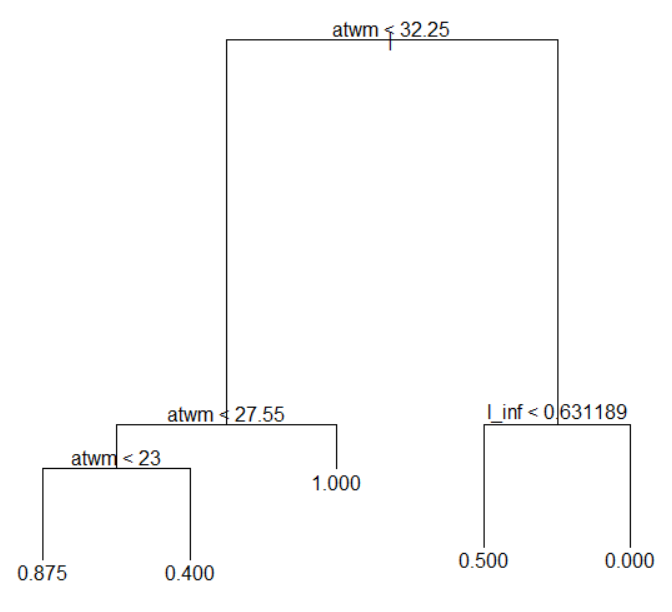I use tree() function in tree package. In this model, "atwm" is the most important variable and l_inf is next. Others are not important.

Let's get accuracy of tree model.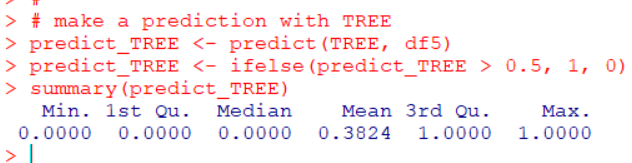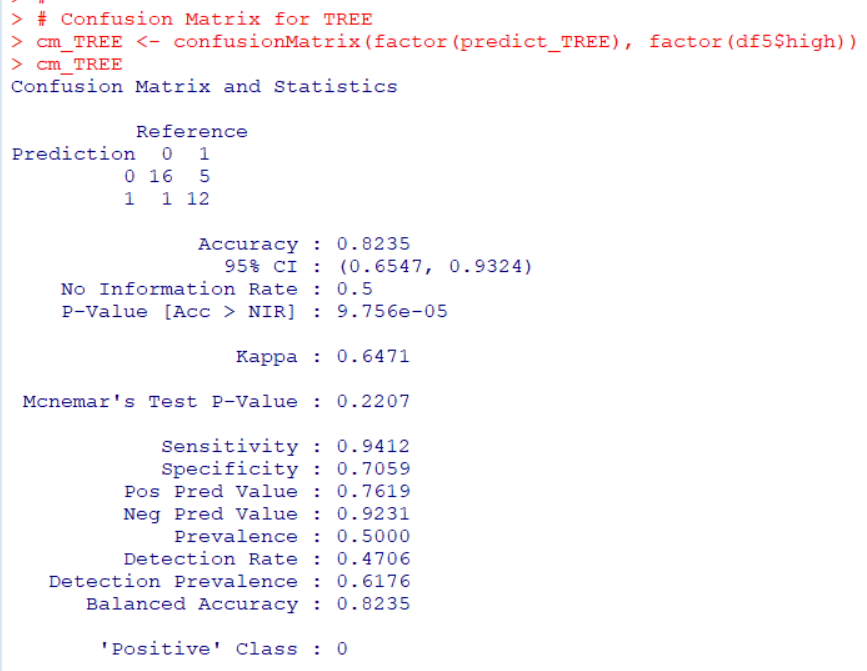Accuracy is 0.8235.

Next, I use k-NN model.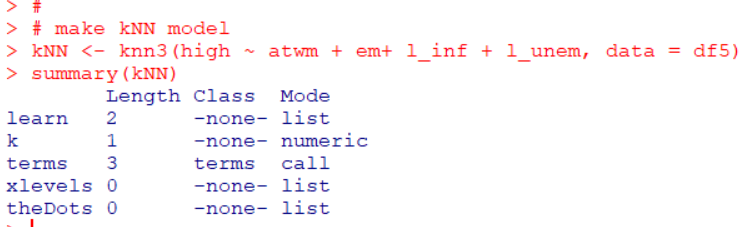I use knn3() function in caret package.

Let's get accuracy of knn.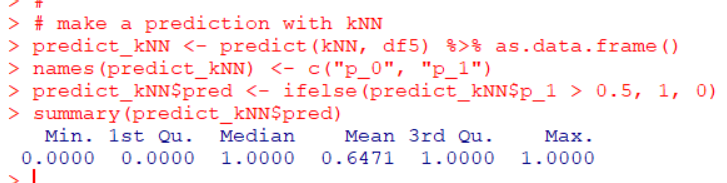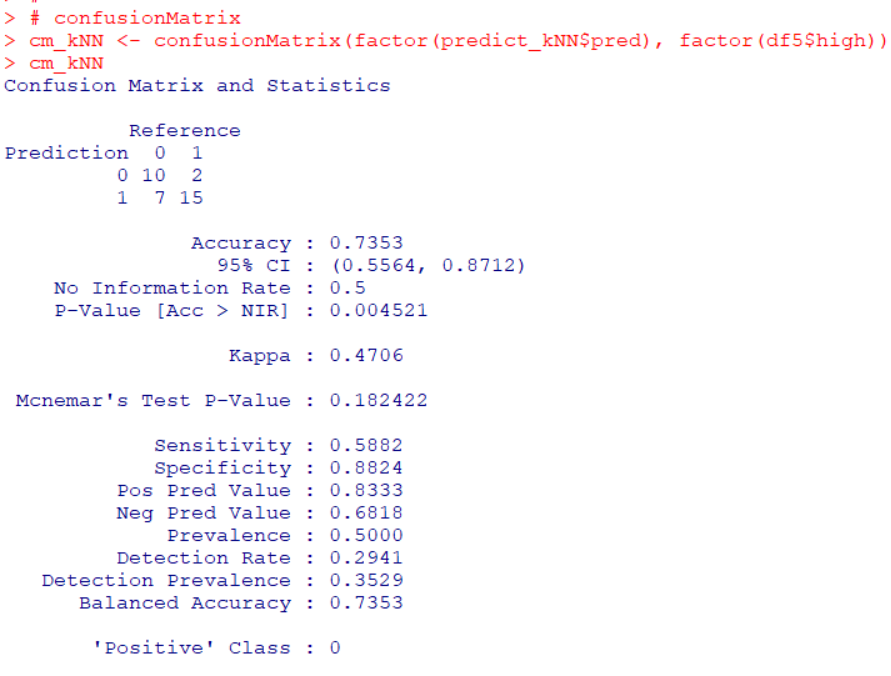Accuracy is 0.7353.

Then, let's compare those methods accuracy.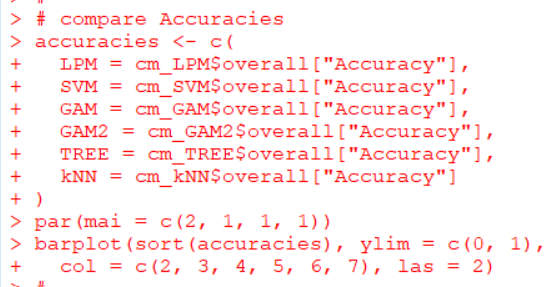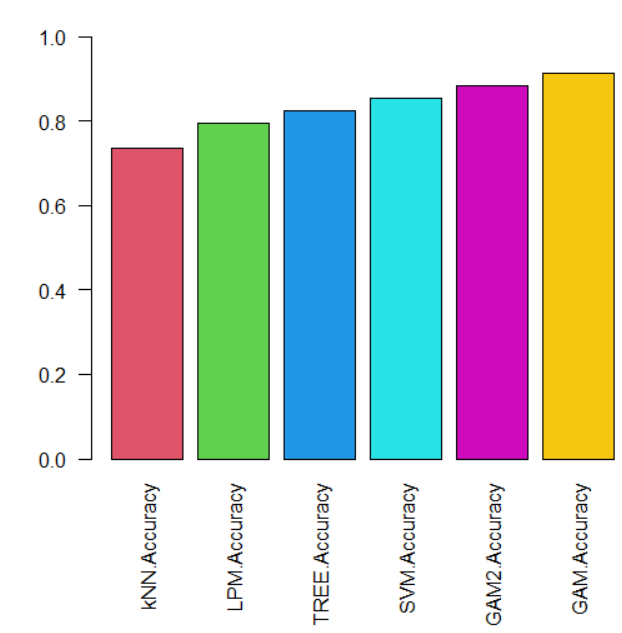GAM model has the highest accuracy.
That's it. Thank you!